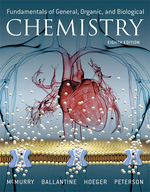×
Get Full Access to Fundamentals Of General, Organic, And Biological Chemistry (Mastering Chemistry) - 8 Edition - Chapter 5.5 - Problem 5.8
Get Full Access to Fundamentals Of General, Organic, And Biological Chemistry (Mastering Chemistry) - 8 Edition - Chapter 5.5 - Problem 5.8

×ISBN: 9780134015187 2044

## Solution for problem 5.8 Chapter 5.5

Fundamentals of General, Organic, and Biological Chemistry (Mastering Chemistry) | 8th Edition

• Textbook Solutions
• 2901 Step-by-step solutions solved by professors and subject experts
• Get 24/7 help from StudySoup virtual teaching assistantsFundamentals of General, Organic, and Biological Chemistry (Mastering Chemistry) | 8th Edition

4 5 1 277 Reviews
21
0
Problem 5.8

Identify the oxidized reactant, the reduced reactant, the oxidizing agent, and the reducing agent in the following reactions:

(a) $$\mathrm{Fe}(s)+\mathrm{Cu}^{2+}(a q) \longrightarrow \mathrm{Fe}^{2+}(a q)+\mathrm{Cu}(s)$$

(b) $$\mathrm{Mg}(s)+\mathrm{Cl}_{2}(g) \longrightarrow \mathrm{MgCl}_{2}(s)$$

(c) $$2 \mathrm{Al}(s)+\mathrm{Cr}_{2} \mathrm{O}_{3}(s) \longrightarrow 2 \mathrm{Cr}(s)+\mathrm{Al}_{2} \mathrm{O}_{3}(s)$$

Text Transcription:

Fe(s) + Cu^2 (aq) arrow right Fe^+2 (aq) + Cu (s)

Mg (s) + CI _2 )g) arrow right MgCI_2 (s)

2 AI (s) + Cr_2O_3 (s) arrow right 2Cr (s) + Ai_2O_3 (s)

Step-by-Step Solution:
Step 1 of 3

Chemistry notes week 1 Underlined= main topic Highlighted= vocabulary Highlighted= important name Bold= important to remember about the topic  Chemistry- the study of matter and the changes in matter  The changes in matter often include these two:  Physical change- A change in matter that does not include changing the chemical composition of the object  Ex. Ripping a piece of paper is a physical change because the chemical composition did not change  Chemical change- a change in matter where the chemical composition is changed after the reaction is complete  Ex. Burning a piece of paper is a chemical change because the paper has become ash and gas  Rusting, burning, and a production of gas are all good signals that a chemical change in occurring  The Scientific Method  Steps:  1. Make an observation  2. Make a prediction/ form a hypothesis, or a guess as to why something happens  3. Test the hypothesis through experimentation  4. Revise or throw out the hypothesis depending on how successful it was  5. Test the revised hypothesis  The scientific method could lead to two things:  Theory- an extensively tested hypothesis that continues to be proved true  The Atom Theory- everything is made of atoms  This theory has been extensively tested and backed up with evidence  Law- Observation that proves to be true by continuous observations

Step 2 of 3

Step 3 of 3

##### ISBN: 9780134015187

This textbook survival guide was created for the textbook: Fundamentals of General, Organic, and Biological Chemistry (Mastering Chemistry), edition: 8. Fundamentals of General, Organic, and Biological Chemistry (Mastering Chemistry) was written by Aimee Notetaker and is associated to the ISBN: 9780134015187. The full step-by-step solution to problem: 5.8 from chapter: 5.5 was answered by Aimee Notetaker, our top Chemistry solution expert on 04/25/22, 03:57PM. The answer to “?Identify the oxidized reactant, the reduced reactant, the oxidizing agent, and the reducing agent in the following reactions: (a) $$\mathrm{Fe}(s)+\mathrm{Cu}^{2+}(a q) \longrightarrow \mathrm{Fe}^{2+}(a q)+\mathrm{Cu}(s)$$ (b) $$\mathrm{Mg}(s)+\mathrm{Cl}_{2}(g) \longrightarrow \mathrm{MgCl}_{2}(s)$$(c) $$2 \mathrm{Al}(s)+\mathrm{Cr}_{2} \mathrm{O}_{3}(s) \longrightarrow 2 \mathrm{Cr}(s)+\mathrm{Al}_{2} \mathrm{O}_{3}(s)$$Text Transcription:Fe(s) + Cu^2 (aq) arrow right Fe^+2 (aq) + Cu (s)Mg (s) + CI _2 )g) arrow right MgCI_2 (s)2 AI (s) + Cr_2O_3 (s) arrow right 2Cr (s) + Ai_2O_3 (s)” is broken down into a number of easy to follow steps, and 67 words. This full solution covers the following key subjects: . This expansive textbook survival guide covers 281 chapters, and 240 solutions. Since the solution to 5.8 from 5.5 chapter was answered, more than 201 students have viewed the full step-by-step answer.

Unlock Textbook Solution